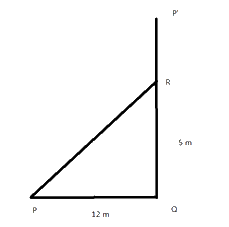# Ex.6.5 Q5 The Triangle and Its Properties - NCERT Maths Class 7

Go back to  'Ex.6.5'

## Question

A tree is broken at a height of $$\rm{}5\, m$$ from the ground and its top touches the ground at $$\rm{}12\, m$$ from the base of the tree. Find the original height of the tree.Video Solution
Triangle & Its Properties
Ex 6.5 | Question 5

## Text Solution

What is known?

Height of the point where the tree broke from the ground is $$5 \rm \,m$$ and distance between the base of the tree and the top of the tree when it touches the ground is $$\rm{}12\, m$$

What is unknown?

Original height of the tree..

Reasoning:

This question is also based on the concept of right-angled triangle and Pythagoras theorem. Suppose $$\rm{}P’Q$$is the height of the tree, as it is mentioned in the question that the tree is broken at a height of $$\rm{}5\,m$$ from the ground. suppose tree is broken from $$R$$ So, consider $$RQ$$ as perpendicular and $$PR$$ as broken part of the treeand, as hypotenuse. Remember, length of $$PR$$ i.e. broken part of the tree will remain same as $$P’R.$$ Now, a right – angled triangle $$PQR$$ is formed, apply Pythagoras theorem and find the length of broken part i.e. $$PR$$. As we must find out the original height of the tree for this, add the length of the broken part and the length where it broke i.e. $$QR.$$

For better visual understanding draw a right-angled triangle and visualise all the parts.

Steps

Let $$P’Q$$ represents the original height of the tree before it broken at $$R$$ and $$RP$$ represents the broken part of the tree.

Triangle $$PQR$$ is right angled at $$Q$$. So, in this triangle, according to Pythagoras theorem,

\begin{align}&{{\left( \text{Hypotenuse} \right)}^2} \\&= \rm{{ }}{{\left(\text {Perpendicular} \right)}^2} + {\rm{ }}{{\left( \text{Base} \right)}^2} \end{align}

\begin{align} {{\left( {PR} \right)}^2} &={{\left( {RQ} \right)}^2} +{{\left( {PQ} \right)}^2}\\{{\left( {PR} \right)}^2} &= \rm{{ }}{{\left( 5 \right)}^2} + {\rm{ }}{{\left( {12} \right)}^2}\\{{\left( {PR} \right)}^2} &= \rm{{ }}25 + 144\\{{\left( {PR} \right)}^2} &= \rm{{ }}169\\PR{\rm{ }} &= \rm{{ }}13{\rm{ }}m\end{align}

Thus, the original height of the tree

\begin{align}&= PR + RQ \\&= \rm{}13\, m + 5\, m\\&= \rm{}18\,\rm{m} \end{align}
Thus, the original height of the tree is $$\rm{}18\, m.$$

Useful Tip:

Whenever you encounter problem of this kind, it is best to think of the concept of a right-angled triangle.

Learn from the best math teachers and top your exams

• Live one on one classroom and doubt clearing
• Practice worksheets in and after class for conceptual clarity
• Personalized curriculum to keep up with school